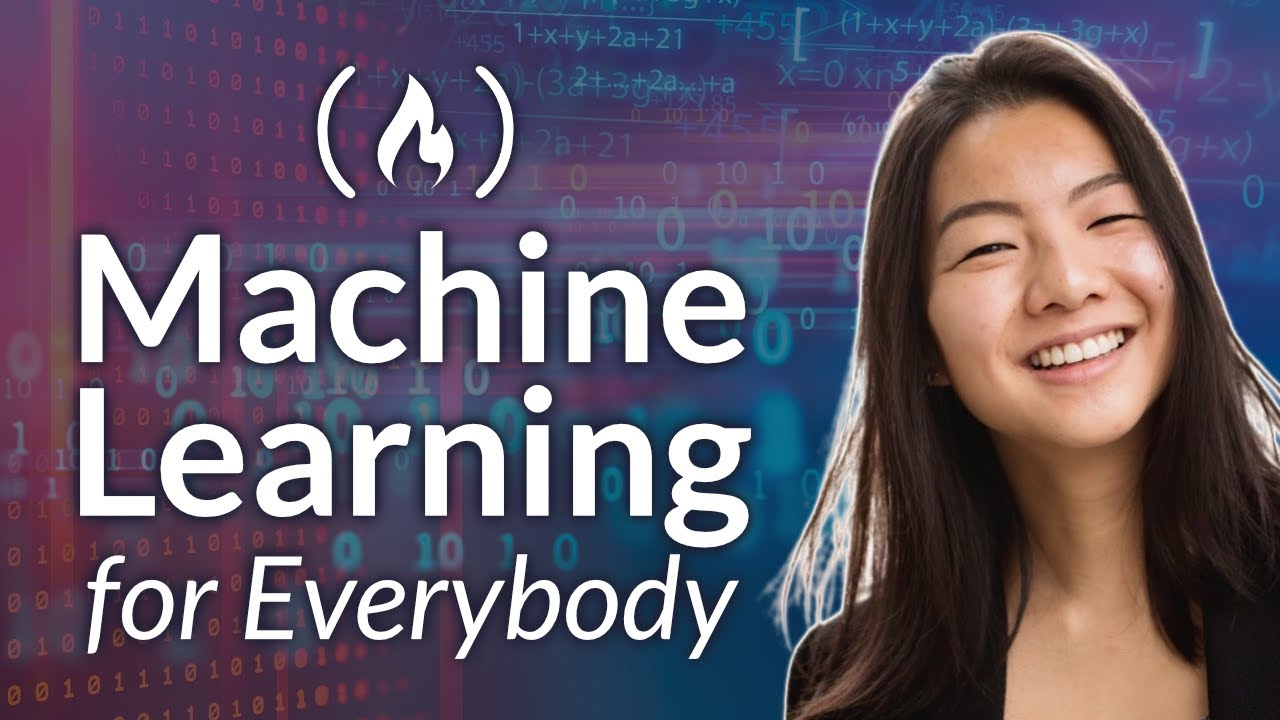# Machine Learning for Everybody – Full CourseLearn Machine Learning in a way that is accessible to absolute beginners. You will learn the basics of Machine Learning and how to use TensorFlow to implement many different concepts.

Learn Machine Learning in a way that is accessible to absolute beginners. You will learn the basics of Machine Learning and how to use TensorFlow to implement many different concepts.

⭐️ Contents ⭐️
⌨️ (0:00:00) Intro
⌨️ (0:00:58) Data/Colab Intro
⌨️ (0:08:45) Intro to Machine Learning
⌨️ (0:12:26) Features
⌨️ (0:17:23) Classification/Regression
⌨️ (0:19:57) Training Model
⌨️ (0:30:57) Preparing Data
⌨️ (0:44:43) K-Nearest Neighbors
⌨️ (0:52:42) KNN Implementation
⌨️ (1:08:43) Naive Bayes
⌨️ (1:17:30) Naive Bayes Implementation
⌨️ (1:19:22) Logistic Regression
⌨️ (1:27:56) Log Regression Implementation
⌨️ (1:29:13) Support Vector Machine
⌨️ (1:37:54) SVM Implementation
⌨️ (1:39:44) Neural Networks
⌨️ (1:47:57) Tensorflow
⌨️ (1:49:50) Classification NN using Tensorflow
⌨️ (2:10:12) Linear Regression
⌨️ (2:34:54) Lin Regression Implementation
⌨️ (2:57:44) Lin Regression using a Neuron
⌨️ (3:00:15) Regression NN using Tensorflow
⌨️ (3:13:13) K-Means Clustering
⌨️ (3:23:46) Principal Component Analysis
⌨️ (3:33:54) K-Means and PCA Implementations